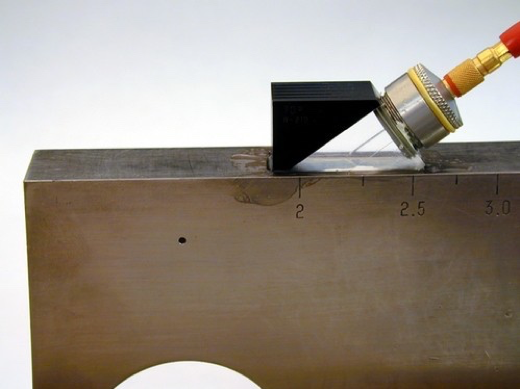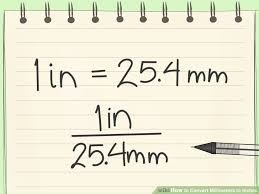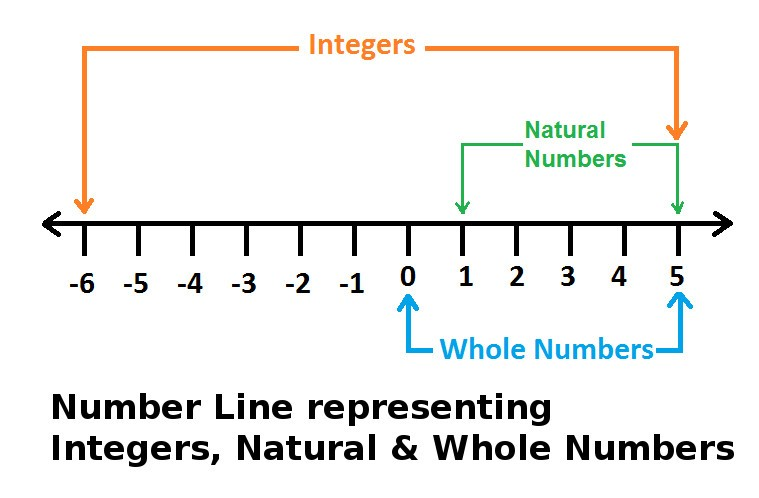# How to Calculate AWS D1.1 Indication Rating?

By: NDT Training - Worldwide | Oct 06, 2020 04:19 AM#### Indication Rating

The “Indication Rating (d)” is the algebraic difference in decibels (dB) between the Indication Level and the Reference Level with Correction for Attenuation as indicated in the following expressions:

Instruments with gain in dB:

Indication Rating => d = a - b - c

Where,

d = Indication Rating (dB)

a = Discontinuity Indication Level (dB)

b = Zero Reference Level (dB)

c = Attenuation factor (dB)

Zero Reference Level "b"

The transducer shall be placed in the position shown and the signal amplitude from the hole (Ø1.5 mm) will be maximized.Adjust the dB in order to obtain a signal from 40 to 60% Full-Screen Height (FSH). This will be used as the Reference Level “b”.

#### Discontinuity Indication Level "a"

When a discontinuity indication appears on the screen, the maximum attainable indication from the discontinuity shall be adjusted to produce a horizontal reference level trace deflection on the screen.

The adjustment shall be made with the calibrated gain control (attenuator), and the instrument reading in decibels shall be used as the “Indication Level "a”.

Increase or decrease the dB until the screen height from the discontinuity is equal to the reference level. Record the dB under Indication Level "a"

#### Attenuation Factor "c"

U. S. Customary Units => The “Attenuation Factor, c” shall be attained by subtracting 1 in. from the sound – path distance and multiplying the remainder by 2.

SI Units => The “Attenuation Factor, c” shall be attained by subtracting 25 mm from the sound – path distance and multiplying the remainder by 0.08.

Inch to SI System Conversion [2 becomes 0.08 when units changed from U.S. Customary to SI]1 inch = 25 mm

=> 1 mm = 1/25 inch = 0.04 inch

Multiplying the remainder by 2

=> 2 * 1 mm = 2 * 0.04 = 0.08

﻿Example (U. S. Customary Units)

If Discontinuity Beam Path = 4 inch

=> Attenuation Factor, c (dB) = [Beam Path - 1 inch] x 2 = [4 inch - 1 inch] x 2 = 3 x 2 = 6 dB

Example (SI Units)

If Discontinuity Beam Path = 100 mm

=> Attenuation Factor, c (dB) = [Beam Path - 25 mm] x 0.08 = [100 - 25] x 0.08 = 75 x 0.08 = 6 dB

It is Always a Whole Number

Attenuation number will be rounded to the nearest whole number.

• If the number is less than 0.5 dB it will be lowered to the nearest whole number.
• If the number is 0.5 dB or greater, it will be increased to the nearest whole number.

Example - 1 (U. S. Customary Units)

If Discontinuity Beam Path = 1.9 inch

=> Attenuation Factor, c (dB) = [Beam Path - 1 inch] x 2 = [1.9 inch - 1 inch] x 2 = 0.9 x 2 = 1.8 dB

=> Hence the Attenuation Factor, c (dB) ~ 2 dB (Rounded to the nearest whole number ~ 2)Example - 2 (U. S. Customary Units)

If Discontinuity Beam Path = 4.1 inch

=> Attenuation Factor, c (dB) = [Beam Path - 1 inch] x 2 = [4.1 inch - 1 inch] x 2 = 3.1 x 2 = 6.2 dB

=> Hence the Attenuation Factor, c (dB) ~ 6 dB (Rounded to the nearest whole number ~ 6)

##### References
1. AWS D1.1/D1.1M: 2015 => Structural Welding Code - Steel
2. Internet Resources